## 图像分割—基于边界的图像分割

### 边缘检测##### 部分常用的边缘检测算子处理效果展示
1. 梯度算子 $\nabla f=\begin{bmatrix} G_x \\ G_y \\ \end{bmatrix}=\begin{bmatrix} \frac{\partial F}{\partial x} \\ \\ \frac{\partial F}{\partial y} \\ \end{bmatrix}$
2. Roberts算子 $\begin{bmatrix} 1 & 0 \\ 0 & -1 \\ \end{bmatrix}\begin{bmatrix} 0 & 1 \\ -1 & 0 \\ \end{bmatrix}$
3. Prewitt算子 $\begin{bmatrix} -1 & 0 & 1 \\ -1 & 0 & 1 \\ -1 & 0 & 1 \\ \end{bmatrix}\begin{bmatrix} 1 & 1 & 1 \\ 0 & 0 & 0 \\ -1 & -1 & -1 \\ \end{bmatrix}$
4. Sobel算子 $\begin{bmatrix} -1 & 0 & 1 \\ -2 & 0 & 2 \\ -1 & 0 & 1 \\ \end{bmatrix}\begin{bmatrix} 1 & 2 & 1 \\ 0 & 0 & 0 \\ -1 & -2 & -1 \\ \end{bmatrix}$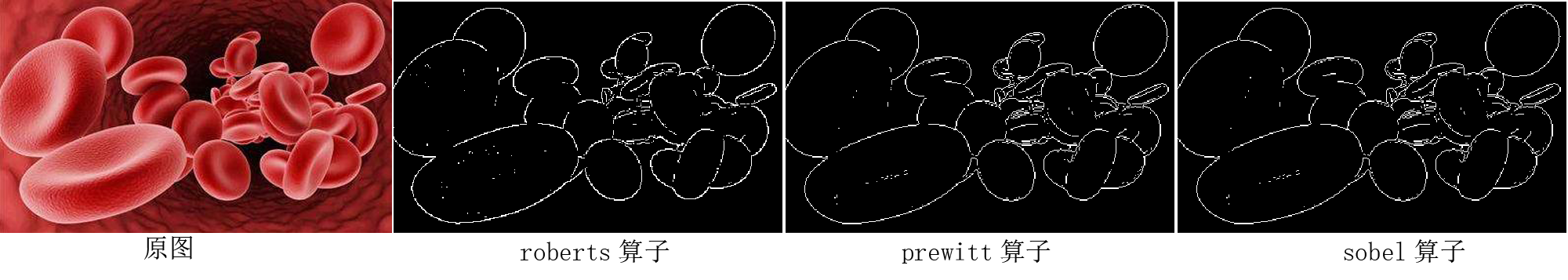### 边缘连接

##### 1、局部处理法

1.1 基本原理：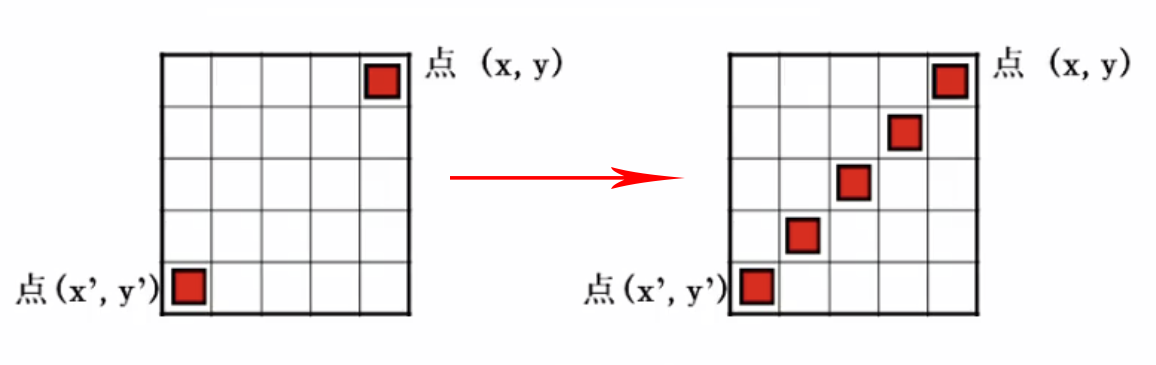• 通过比较梯度强度确定两个点之间的连接性：
对于点 $(x',y')$，判断其是否与领域内的点 $(x,y)$相似，当 $|\nabla f(x,y)-\nabla f(x',y')|\leqslant T$时可以判定两个像素之间的梯度强度相近。
• 通过比较梯度方向确定两个点之间的连接性：
对于点 $(x',y')$，判断其是否与领域内的点 $(x,y)$的方向角相似，当 $|\alpha(x,y)-\alpha(x',y')|\leqslant T$时可以判定两个像素之间的有相互连接的趋势。

1.2 边缘跟踪：

• 光栅跟踪
采用电视光栅行扫描顺序，结合阈值检测，对遇到的像素进行分析从而确定是否为边缘的跟踪方法，具体步骤如下：
(1) 选取一个较大的检测阈值 $d$，对图像第一行进行阈值化。大于 $d$的像素作为对象点，并作为下一步跟踪的起始点
(2) 选取一个较小的跟踪阈值 $k$，例如灰度差阈值
(3) 扫描下一行像素（像素 $(i,j)$的下一行像素 $(i+1,j-1)$ $(i+1,j)$ $(i+1,j+1)$为跟踪邻域），凡与上一行对象点相邻接的像素灰度差小于 $k$的且值最小的点接受为新对象点，反之去除
(4) 对未被接受为对象点的其他各行像素再次从步骤(1)开始重复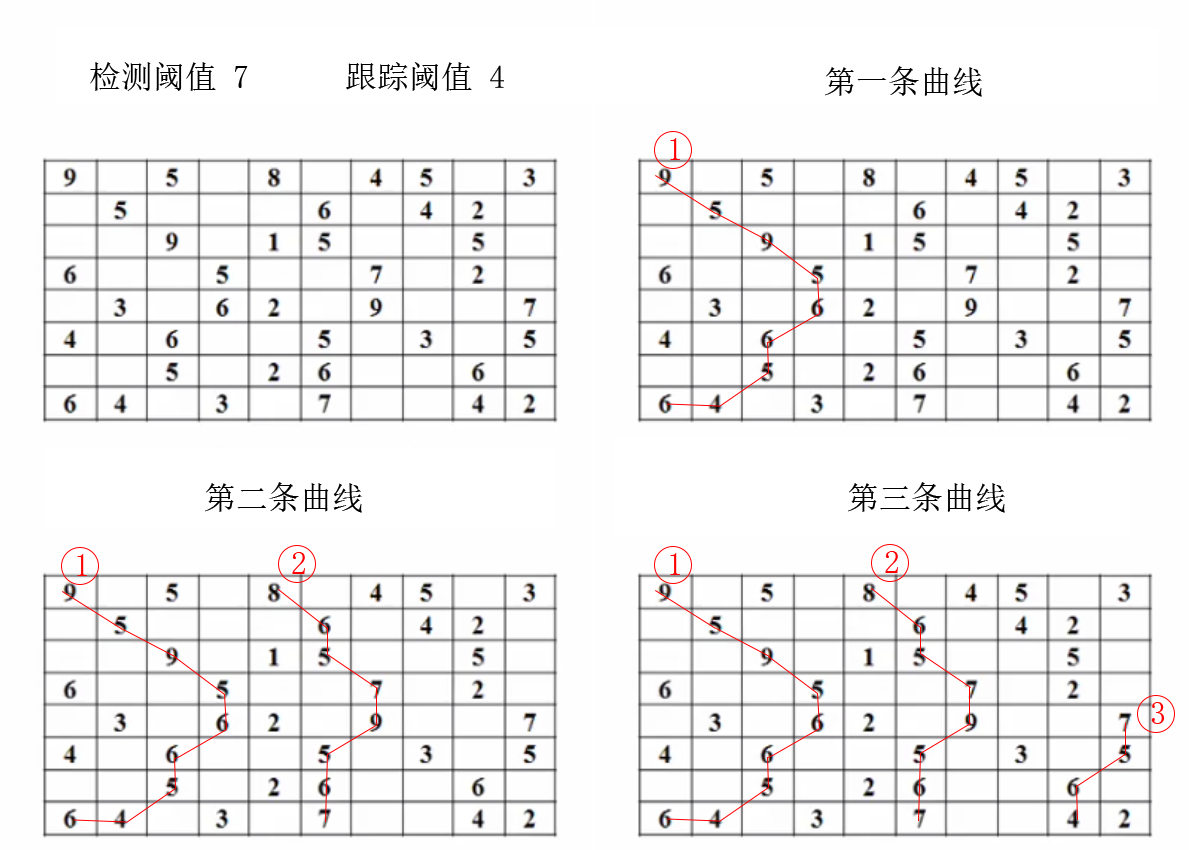• 全向跟踪
全向跟踪是指跟踪方向可以是任意方向，并且有足够大的跟踪距离的跟踪方法。是一种改变了邻域定义和跟踪准则的光栅跟踪法，其跟踪步骤和原则与光栅跟踪法一致
(1) 邻域：选取适当的、能进行全向跟踪的邻域，例如八邻域等
(2) 跟踪准则：选择一个适当的跟踪准则，如灰度阈值、对比度和空间距离等
##### 2、Hough变换

Hough变换检测直线算法

1. 在算法的实际使用过程中，为了避免出现无穷大，常用极坐标形式代替直角坐标形式。此时，参数平面就为 $\rho\theta平面$，而不是直角坐标下的 $ab$平面：
$x\cos\theta+y\sin\theta=\rho$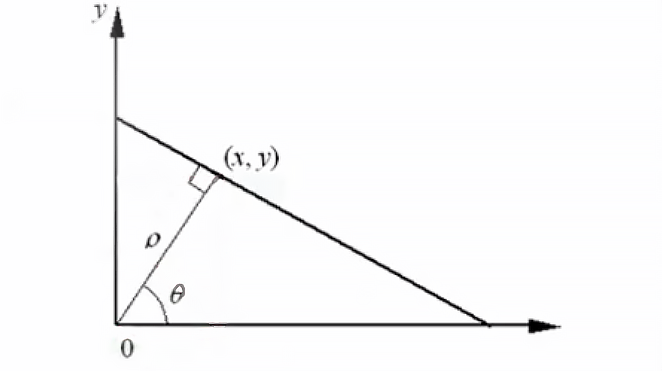1. 创建交点累加器或交点统计直方图，找出相交线最多的参数空间的点
2. 找出该点对应的未知数平面的直线线段，即检测完成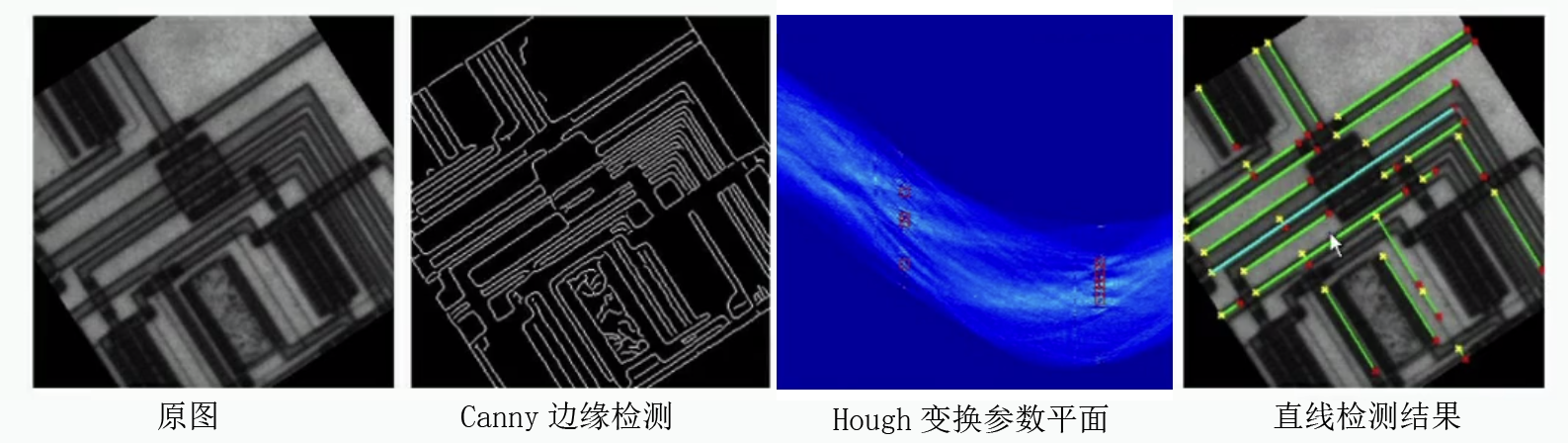Hough变换的扩展

1. 检测圆
将Hough变换应用到对圆的检测上时，其参数方程变换为 $(x-c_1)^2+(y-c_2)^2=c_3^2$此时需要三个参数的参数空间
2. 检测椭圆
参数方程设为 $\frac{(x-x_0)^2}{a^2}+\frac{(y-y_0)^2}{b^2}=1$微分后有 $\frac{x-x_0}{a^2}+\frac{y-y_0}{b^2}·\frac{\text{d}y}{\text{d}x}=0$微分后的方程只有三个独立参数，只需从 $(a,b,x_0,y_0)$中选择三个参数进行检测即可